edHelper.com Life Skills Writing Checks Math Worksheets Money Worksheets, Printables, Activities, and Lesson Plans 3rd Grade MoneyWorksheets, Lessons, and Printables

Math Learning Center: Counting Coins and Money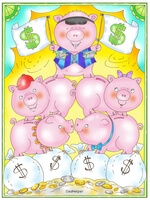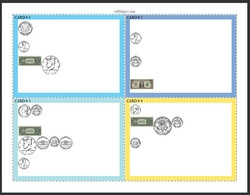U.S. Money: Coins only (Grades 3-6) U.S. Money: Bills only (Grades 3-6) U.S. Money: At least one coin and one bill on each card (Grades 3-6) U.S. Money: Mix (Grades 3-6)

Euros - Math Learning Center: Counting Coins and MoneyEuros: Coins less than 100 Euros (Grades 3-6) Euros: Coins at least 100 Eurus (Grades 3-6) Euros: Mix of small and large coins (Grades 3-6) Euros: Mix (Grades 3-6)

Canadian Currency - Math Learning Center: Counting Coins and MoneyCanadian Currency: Coins less than 100 cents (Grades 3-6) Canadian Currency: Coins at least 100 cents (Grades 3-6) Canadian Currency: Mix of small and large coins (Grades 3-6) Canadian Currency: Mix (Grades 3-6)

3-Digit Subtraction
2-Digits from 3-Digits
Subtract money
3-Digits
Subtract money

3-Digit Addition and Subtraction
3-digit addition and subtraction of money
Problem solving: Money word problems

Write in decimal form (money graphics)
Write in decimal form (sixty-five dollars and one cent)
Write in decimal form (4 one-dollar bills, 3 quarters, 1 dime)
Compare (money graphics)
Compare
Show amount using fewest number of coins (20 to 99 cents)
Show amount using fewest number of coins (20 to 99 cents; fill-ins have mixed order)
Show amount using fewest number of coins (\$0.20 to \$4.99)
Show amount using fewest number of coins (\$0.20 to \$19.99)
Make change using the given number of coins (20 to 99 cents)
Make change using the given number of coins (\$0.20 to \$4.99)
Make change using the given number of coins (\$0.20 to \$19.99)
Compare (\$1.59 ____ \$1.95)
Round to the nearest dollar
Round to the nearest ten dollars
Round to the place of the underlined digit
Problem solving
Money Mixed Review

Logic Puzzles: Coins - Easier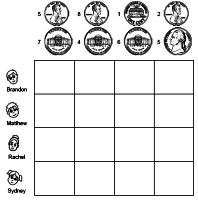Pennies and nickels Nickels and dimes Pennies, Nickels, or Dimes (each key will use pick two coins)

Logic Puzzles: Coins - Greater and Less than Clues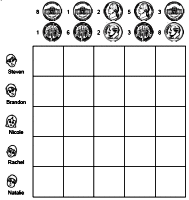Pennies and nickels Nickels and dimes Pennies, Nickels, or Dimes (each key will use pick two coins)

Logic Puzzles: Coins - Comparing Total Value of Coins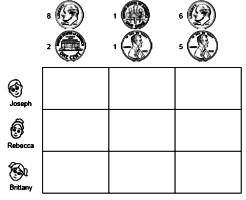Pennies and nickels Nickels and dimes Pennies, Nickels, or Dimes (each key will use pick two coins)

Money

Have a suggestion or would like to leave feedback?